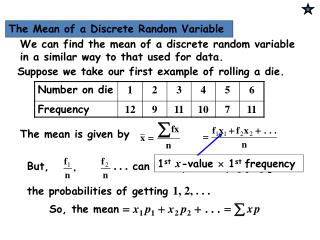DownloadDownload Presentation1 st x -value  1 st frequency

# 1 st x -value  1 st frequency

Télécharger la présentation## 1 st x -value  1 st frequency

- - - - - - - - - - - - - - - - - - - - - - - - - - - E N D - - - - - - - - - - - - - - - - - - - - - - - - - - -
##### Presentation Transcript

1. But, . . . can be replaced by , . . . the probabilities of getting 1, 2, . . . 1stx-value  1st frequency So, the mean The Mean of a Discrete Random Variable We can find the mean of a discrete random variable in a similar way to that used for data. Suppose we take our first example of rolling a die. The mean is given by

2. pronounced “mew” or, more often, replacing p by , Notation for the Mean of a Discrete Random Variable When dealing with a model, we use the letter m for the mean (the greek letter m). We write Instead of m, we can also write E(X). This notation comes from the idea of the mean being the Expected value of the r.v. X.

3. pronounced “mew” or, more often, replacing p by , Notation for the Mean of a Discrete Random Variable When dealing with a model, we use the letter m for the mean (the greek letter m). We write Instead of m, we can also write E(X). This notation comes from the idea of the mean being the Expected value of the r.v. X. ( Think of this as being what we expect to get on average ).

4. e.g. 1. A random variable X has the probability distribution x P (X = ) Find (a) the mean of X. Tip: Always check that your value of the mean lies within the range of the given values of x. Here, or 5·25, does lie between 1 and 10. Solution: (a) mean,

5. Expectation of a continuous random variable • E(X) = m = This formula is similar to that used in discrete random variables E(X) = xP(X = x) Replace • Ex1 Find the expected value of E(X) = m = The boundaries are 16 and  as x can take any value greater than 16

6. E(X) = m = E(X) = A= 0 as = 0 if x =  A16= = -26 Area = 0 - -26 = 26 So the mean = 26

7. Symmetry If a function is symmetrical then the expected value lies on the line of symmetry Ex2 From the sketch the line of symmetry clearly lies on x = 2 So E(X) = 2

8. For a frequency distribution, the formula is Replacing by etc. gives Variance of a Discrete Random Variable The variance of a discrete random variable is found in a similar way to the one we used for the mean.

9. But we must replace by So, The variance of X is also written as Var(X).

10. e.g. 1 Find the variance of X for the following: x P(X = x ) Solution: We first need to find the mean, m :

11. Variance of a continuous random variable Var(X) = 2= This formula is similar to that used in discrete random variables Var(X) = x2P(X = x) – mean2 Replace Ex2 From the example above E(X) = 2 So m = 2

12. Var(X) = 2 = A4 = 4.8 A0 = 0 Area = 4.8 - 0 = 4.8 So Var(X) = • = 4.8 - 22 = 0.8 S.D = = 0.9